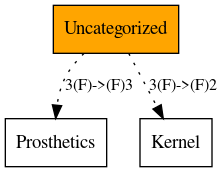Home   Package List   Routine Alphabetical List   Global Alphabetical List   FileMan Files List   FileMan Sub-Files List   Package Component Lists   Package-Namespace Mapping
Package: Uncategorized

# Package: Uncategorized

## Dependency Graph## Non-FileMan Globals, Total: 121

 ^\$DI( ^\$E("WINDOW" ^\$EVENT("ELEMENT" ^\$EVENT("OK" ^\$EVENT("WINDOW" ^\$JOB( ^\$R( ^\$ROUTINE( ^\$W("MESSAGE" ^\$W("ZISGDEV" ^\$W("ZISGHFS" ^\$W("ZISGMT" ^\$W("ZISGSDP" ^\$W("ZISGSPL" ^\$W("ZISGTRM" ^\$WINDOW("ZISGDEV" ^%IS( ^%IS(0 ^AKF("FAX" ^AKF("FAXG" ^AKF("FAXR" ^ANUSB(626140 ^APCCCTRL(0 ^APMM(99.3 ^AUPDVIMM("AD" ^AUPDVIMM("ADA" ^AUPDVIMM("ADP" ^AUPDVIMM("B" ^AUPDVIMM("C" ^AUPDVIMM("DD" ^BTMP(364.1 ^BWAGDF ^BWCUR ^BWDIAG ^BWEDC ^BWLET ^BWMAMT ^BWMGR ^BWNOT ^BWNOTO ^BWNOTP ^BWNOTT ^BWP ^BWPCD ^BWPLOG ^BWPN ^BWPR ^BWRADX ^BWSITE ^BWSNAP ^DSIPPOA( ^DSIPPOA("B" ^DSIPPRV ^DSIPPRV("B" ^DTGHD("A" ^FILE(100 ^FILE(101.41 ^FILTERS("B" ^GEK("SETVAL" ^GMR( ^GMR(120.83 ^GMR(121 ^GMR(121.1 ^GMR(121.2 ^GMR(121.99 ^GMR(128 ^IMV(301.93 ^JADREJ( ^JADUTIL("AWP UPDATE" ^KMTMP("KMPDT" ^KUMDG3 ^KUMDG4 ^NDFK( ^NDFK(5000 ^NDFK(5000.2 ^NDFK(5000.506 ^NDFK(5000.508 ^NDFK(5000.509 ^NDFK(5000.56 ^NDFK(5000.561 ^NDFK(5000.93 ^NTS(2050 ^NTS(2050.2 ^POLCCOM( ^POLCOM( ^PS( ^PS("52." ^PS(50.9 ^PS(53.35 ^PSTRAN("B" ^QA( ^QA(745.2 ^QA(745.5 ^QA(752.5 ^REM("M2MCL" ^ROUTINE( ^ROUTINE("" ^ROUTINE("R1ACSX" ^RWF("PATCH" ^RWF(\$J ^SPOOL( ^SPOOL(0 ^STATS( ^SYS( ^SYS("BLKCOL" ^SYS("CONFIG" ^SYS("UCI" ^SYS(0 ^TRAX( ^TRAX("A" ^TRAX("B" ^TRAX("C" ^UTILTIY(\$J ^UTL("STPCODE" ^XBD(9.6 ^XHLP("D" ^XOB( ^XOB(18.08 ^YKTL( ^ZCONV( ^ZLX(

## Routines, Total: 61

 \$& \$&ZLIB \$&ZLIB.%CRELOG \$&ZLIB.%DISABLCTRL \$&ZLIB.%GETDVI \$&ZLIB.%GETJPI \$&ZLIB.%GETSYM \$&ZLIB.%PARSE \$&ZLIB.%SETSYM \$&ZLIB.%SPAWN %CLOSE %ET %apiOBJ %dsselect @( A6ADOC8 AAH9UF39 AKFAX0 AKFAXD APCDMSR1 AVJLES AYILOCKH BDGF2 BEHOENPC BEHOTIU BEHOVM BIUTL5 CVERCVT DSSOHLC FOIAPRD KBANTCLN MPIDIRQ MPIRPC MPRCHK MUSMCR1 MUSMCR2 MUSMCR3 RGED RGEDBRS RGWBSVR ROUTINE TEST VIARPC3 WPSEFM WPSEHELP WPSEPHR1 WWWMAL83 X XOBTPST XOBTWU ZFOO ZJFOO ZSLOT ZXML3CRC ZXMLBMP ZXMLBMP1 ZZHLGHB1 ZZRCSUM ZZVPS pvPostInstall